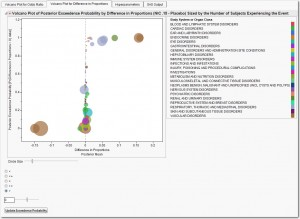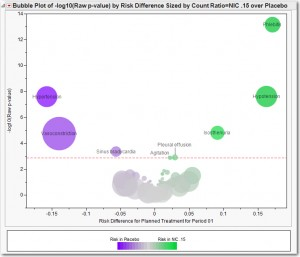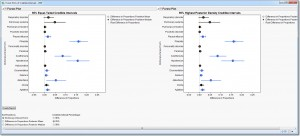Analyzing adverse events using Bayesian hierarchical models

You may be asking yourself… “Two Bayesian posts in a row? What is going on?”

Though my statistical training focused on Frequentist methodologies, I am a big believer in using whatever tools help me gain insight into the statistical problem I happen to be focusing on at the moment. Frequentist or Bayesian, it makes no difference. In fact, because I'm a biostatistician, my appreciation of these two schools of statistical inference makes complete sense.

It is a common misunderstanding that biostatistics focuses on the statistical analysis of medical or biological data. Nothing could be further from the truth.  Let’s dissect the word “biostatistics.” According to the dictionary, “bi-“ means “two.” So clearly, “bi-“ and “statistics” refers to the two major inferential paradigms of modern statistics. What does the “o” stand for? It stands for “oh, how I love.”

So let’s review:

bi                   “Bayesian or Frequentist”

o                     “oh, how I love”

statistics       “statistics”

So biostatistics means “Bayesian or Frequentist, oh, how I love statistics.”

Ramblings aside, today we are going to talk about the analysis of adverse events using Bayesian hierarchical models. It is no surprise that the analysis of adverse events, and the analysis of safety endpoint in general, tend to involve a large number of comparisons between two treatment arms. Further, many adverse events occur infrequently. Finally, typical analysis of adverse events ignores how events may be related to one another.

To address these issues, Berry & Berry (2004) define a three-level hierarchical mixture model that:

1. Analyzes all events simultaneously. Inference is based on summarizing MCMC samples from the posterior distribution.
2. Allows related AEs to borrow strength from one another (based upon system organ class membership, say).
3. Modulates extreme results, possibly due to the rarity of some events.
4. The AE Bayesian Hierarchical Model Analytical Process (AP) fits the Berry & Berry (2004) model in JMP Clinical 4.1 using PROC MCMC to compare the treatment-emergent adverse event profile of two treatment arms. There are options to specify the number of burn-in samples and posterior samples, the thinning rate and the number of independent Markov chains. Further, users can alter the assumed hyperparameter values suggested in Berry & Berry (2004).Figure 1 summarizes the adverse event analysis of Nicardipine versus Placebo using a Bayesian version of a volcano plot (Figure 2). In lieu of the –log10(p-value) for the y-axis, we use the posterior exceedence probability, which is initially defined as p[difference in proportions > 0|data] (or odds ratios could be analyzed instead). For each adverse event, this probability is calculated as the number of posterior samples where the difference in proportions (Nicardipine – Placebo) is > 0 divided by the total number of posterior samples (here:  10,000). This can be interpreted as the probability that Nicardipine has excess risk for an event compared to Placebo. The user is free to alter the definition of the posterior exceedence probability and redraw the volcano plot.The x-axis is similar to the Frequentist volcano plot, except that we use the posterior mean of the difference in proportions (which is just the average of all posterior samples). Bubbles are sized so that the area of the bubble is proportional to the total number of subjects experiencing the event across both treatments combined. Color indicates the system organ class to which events belong. It is straightforward to observe that several vascular disorders, respiratory and psychiatric disorders show increased risk for Nicardipine.

Figure 3 contains Forest Plots that summarize the equal-tailed and HPD posterior credible intervals for all events where p[difference in proportions > 0|data] > 0.80. Using the equal-tailed intervals, we can see there are several events that appear to have higher risk for Nicardipine: Isosthenuria, Phlebitis, Pleural effusion, Hypotension and Agitation. These events also stand out in Figure 2.

However, the Bayesian model highlights additional events: Atelectasis (collapse of part of the lung) , Delirium and Disorientation. Atelectasis was experienced by 86 (19.2%) and 63 (13.8%), Delirium by 6 (1.3%) and 0 and Disorientation by 8 (1.8%) and 3 (0.7%) subjects for Nicardipine and Placebo, respectively. The raw and FDR-adjusted p-values for Atelectasis  were 0.0293 and 0.4100, Delirium were 0.0132 and 0.2876, and Disorientation were 0.1222 and 0.5416, respectively. Redefining the exceedence probability and examining events where p[difference in proportions < 0|data] > 0.80 shows an increased risk of Hypertension and Vasoconstriction for Placebo (which agrees with the analysis in Figure 2). However, Sinus Bradycardia shows no important difference between treatments.  This is likely due to the fact that there are 12 other events in the Cardiac Disorders system organ class with no treatment effects.  Ideally, the Bayesian analysis should assess the sensitivity of the results to different prior assumptions, but we have identified some additional adverse events between the treatments that require greater scrutiny.Alternatively, a model that adjusts for subject-exposure can be used to analyze adverse events in JMP Clinical (Xia, Ma & Carlin, 2010). For subjects with an event, their exposure is calculated as the time from the start of dosing until their first event. The exposure for subjects without an event is computed as their entire time on study.

I'll be discussing these methods in further detail at SAS Global Forum. Feel free to review my paper here.

Article Tags